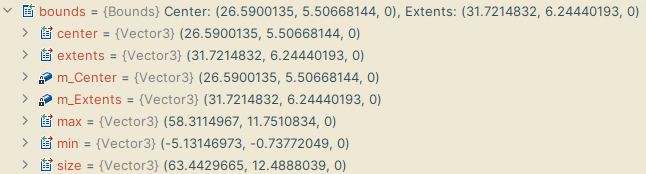# World Coordinates of BoxCollider2D?

Hi how can i calculate the world coordinates of all 4 corners of a boxCollider2D in separate 4 vector3 variables

in PolygonCollider2D we have something like ‘points’ which is local coordinate and then we transform it into world coordinate

my end result is world coordinate so even if i can have local coordinates of boxCollider2D that would be great because i can then just convert it

There are a few ways to do this.

You can get the Bounds of the collider, and use Bampf’s answer here to extract the points of the bounds (that answer is tailored to 3D but the idea is the same).

Or you can use TransformPoint with the collider’s center value to get a world position, and use its size value to get x and y positions based on half the width and height from that world version of the center point.

EDIT:
Here’s an example

``````BoxCollider2D collider = (BoxCollider2D) gameObject.collider2D;

Vector2 size = collider.size;
Vector3 centerPoint = new Vector3( collider.center.x, collider.center.y, 0f);
Vector3 worldPos = transform.TransformPoint ( collider.center );

float top = worldPos.y + (size.y / 2f);
float btm = worldPos.y - (size.y / 2f);
float left = worldPos.x - (size.x / 2f);
float right = worldPos.x + (size.x /2f);

Vector3 topLeft = new Vector3( left, top, worldPos.z);
Vector3 topRight = new Vector3( right, top, worldPos.z);
Vector3 btmLeft = new Vector3( left, btm, worldPos.z);
Vector3 btmRight = new Vector3( right, btm, worldPos.z);
``````

This assumes the script is on the object with the collider (for fetching it), but what you do with the values and where you store them would be up to you.

EDIT 2: Rect example
If your collider is not going to change shape, only position, I’d say you should store info in a rect value that you simply change the center to match the world center of the collider. Then you can use `xMin` / `xMax` and `yMin` / `yMax` to quickly fetch the relative x and y positions of the corners, as follows:

``````BoxCollider2D collider = (BoxCollider2D) gameObject.collider2D;

Vector2 size = collider.size;
Vector3 centerPoint = new Vector3( collider.center.x, collider.center.y, 0f);

Vector3 worldPos = transform.TransformPoint ( collider.center );

Rect rect = new Rect(0f, 0f, size.x, size.y);
rect.center = new Vector2(worldPos.x, worldPos.y);

Vector3 topLeft = new Vector3( rect.xMin, rect.yMax, worldPos.z);
Vector3 topRight = new Vector3( rect.xMax, rect.yMax, worldPos.z);
Vector3 btmLeft = new Vector3( rect.xMin, rect.yMin, worldPos.z);
Vector3 btmRight = new Vector3( rect.xMax, rect.yMin, worldPos.z);
``````

Necro Edit 3:
In response to a recent comment, I’d like to add a third alternative that is really like the first one but more flexible to z rotation. The main difference is that instead of immediately getting the world point of the collider and simply adding the x / y size (not accounting for rotation), you fetch the world point of each of the local points individually in local space (which does account for rotation).

``````BoxCollider2D collider = (BoxCollider2D) this.gameObject.GetComponent<Collider2D>();

float top = collider.offset.y + (collider.size.y / 2f);
float btm = collider.offset.y - (collider.size.y / 2f);
float left = collider.offset.x - (collider.size.x / 2f);
float right = collider.offset.x + (collider.size.x /2f);

Vector3 topLeft = transform.TransformPoint (new Vector3( left, top, 0f));
Vector3 topRight = transform.TransformPoint (new Vector3( right, top, 0f));
Vector3 btmLeft = transform.TransformPoint (new Vector3( left, btm, 0f));
Vector3 btmRight = transform.TransformPoint (new Vector3( right, btm, 0f));
``````

For performance and efficiency, I advise caching these values when you can instead of always calculating them, including caching ‘transform’ and `gameObject` if you are going to be calling this often.

In case this helps future visitors, here’s an alternative to Alex Slayden’s techniques above. This method successfully gives you the 4 points/vertices/corners of a `BoxCollider2D` in world space and handles x/y/z rotation and scale.

``````// Assign the collider in the inspector or elsewhere in your code
BoxCollider2D box;

void GetBoxCorners() {

Transform bcTransform = boxC.transform;

// The collider's centre point in the world
Vector3 worldPosition = bcTransform.TransformPoint(0, 0, 0);

// The collider's local width and height, accounting for scale, divided by 2
Vector2 size = new Vector2(box.size.x * bcTransform.localScale.x * 0.5f, box.size.y * bcTransform.localScale.y * 0.5f);

// STEP 1: FIND LOCAL, UN-ROTATED CORNERS
// Find the 4 corners of the BoxCollider2D in LOCAL space, if the BoxCollider2D had never been rotated
Vector3 corner1 = new Vector2(-size.x, -size.y);
Vector3 corner2 = new Vector2(-size.x, size.y);
Vector3 corner3 = new Vector2(size.x, -size.y);
Vector3 corner4 = new Vector2(size.x, size.y);

// STEP 2: ROTATE CORNERS
// Rotate those 4 corners around the centre of the collider to match its transform.rotation
corner1 = RotatePointAroundPivot(corner1, Vector3.zero, bcTransform.eulerAngles);
corner2 = RotatePointAroundPivot(corner2, Vector3.zero, bcTransform.eulerAngles);
corner3 = RotatePointAroundPivot(corner3, Vector3.zero, bcTransform.eulerAngles);
corner4 = RotatePointAroundPivot(corner4, Vector3.zero, bcTransform.eulerAngles);

// STEP 3: FIND WORLD POSITION OF CORNERS
// Add the 4 rotated corners above to our centre position in WORLD space - and we're done!
corner1 = worldPosition + corner1;
corner2 = worldPosition + corner2;
corner3 = worldPosition + corner3;
corner4 = worldPosition + corner4;

}

// Helper method courtesy of @aldonaletto
Vector3 RotatePointAroundPivot(Vector3 point, Vector3 pivot, Vector3 angles) {
Vector3 dir = point - pivot; // get point direction relative to pivot
dir = Quaternion.Euler(angles) * dir; // rotate it
point = dir + pivot; // calculate rotated point
return point; // return it
}
``````

Collider already has an attribute that could be helpful for this, named “bounds”.Then, the code below to get min, max of X and Y.

``````var boxCollider2D = worldConfinement.GetComponent<BoxCollider2D>();
var bounds = boxCollider2D.bounds;

maxX = bounds.max.x;
minX = bounds.min.x;
maxY = bounds.max.y;
minY = bounds.min.y;
``````